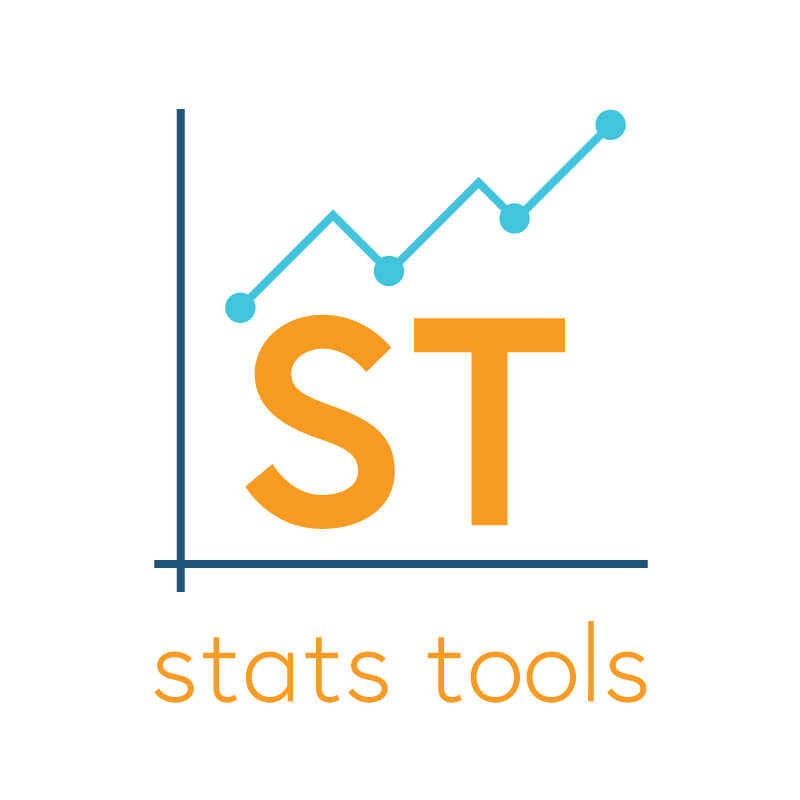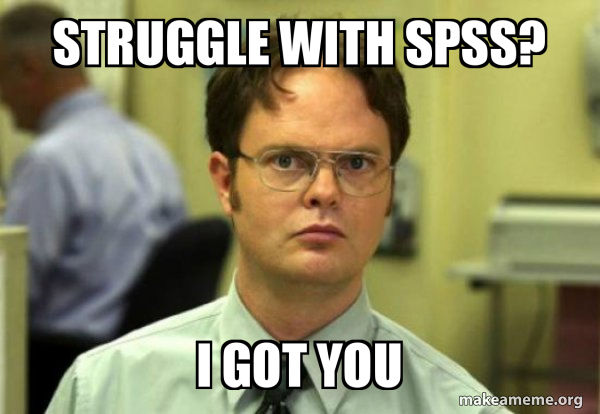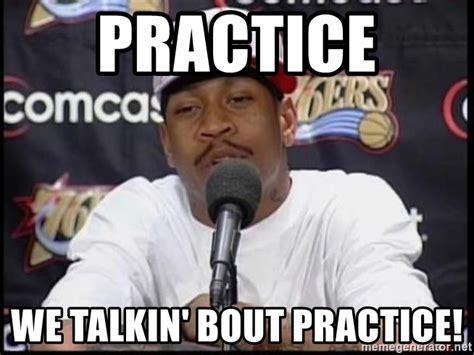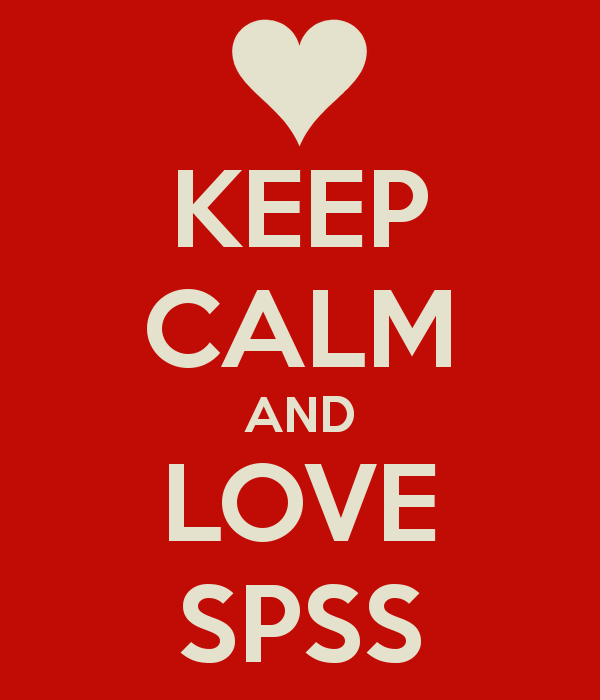# SPSS## Resources: Stats of Doom

A comprehensive site created by Dr. Erin Buchanan offering lecture materials and videos for a full range of statistics concepts covered in a basic/introductory course (descriptives, graphs, z-scores, t-tests, ANOVA, correlation, regression, chi-square, etc.). Coverage also includes materials for several statistics packages (e.g., SPSS, JASP, R) Here is a link for a basic course. There …## Student Guide to SPSS and APA Style Writing (v.12.0)

This guide (created by Gary Lewandowski; Twitter: @LewandowskiPhD) is a free resource that can substitute for the APA Manual and SPSS guides. The Student Guide leads students through data analysis in SPSS with step-by-step instructions and screenshots for setting up the analyses and interpreting the output. For each analysis, students also see an example SPSS …## Video: One-Way ANOVA (SPSS for Beginners)

Video (11:19) from Research by Design described as follows, “Using the same example from the Wizard of Oz involving Munchkins and wicked witches in various regions that we used learning ANOVA by hand, we are going to learn about conducting a one-way ANOVA using SPSS. We will create the dataset in SPSS, conduct a one-way …## Video: Chi-Square (SPSS for Beginners)

Video (7:37) from Research by Design described as follows, “We are now going to learn how to calculate a one-way chi-square in SPSS. This is the House of Pigs Chi Square, using the dataset BuildingPermits.sav. A house inspector randomly samples building permits pulled by 70 little pigs who were building houses, to determine whether a …## Video: Independent-Samples t-test (SPSS for Beginners)

Video (8:11) from Research by Design and Dr. Todd Daniel, described as follows, “Another way of measuring the difference between two samples is to compare two unrelated groups or participants or samples. In this design, you measure two groups one time; in contrast, the previous paired test measured the same sample two times. With independent …## Video: One Sample and Paired-Samples t-test (SPSS for Beginners)

Video (6:02) from Research by Design and Dr. Todd Daniel, described as follows, “When we calculate the mean of a sample, we can then use a one-sample t test to compare that sample mean to another mean, such as a mean from a population, a normative group, or another known value (mean). The “one sample” …## Video: Correlation (SPSS for Beginners)

Video (9:52) from Research by Design and Dr. Todd Daniel, described as follows, “Using the dataset we created in the first video, we now explore the relationship between our two scale-level variables: height and weight. We will use Pearson’s correlation to measure this relationship. We will also learn about a point biserial correlation with gender.” …## Video: Frequencies and Descriptive Statistics (SPSS for Beginners)

Video (14:01) from Research by Design and Dr. Todd Daniel, described as follows, “Having learned how to create a variable, you are ready to begin entering data. Dr. Daniel begins with descriptive statistics (numbers that describe our data) and shows you how to do some basic data cleaning and data exploration with the Frequencies command …## Video: Introduction to SPSS & Creating Variables (SPSS for Beginners)

Video (10:15) from Research by Design and Dr. Todd Daniel, described as follows, “Are you ready to learn how to use SPSS for your introductory statistics class? You have come to the right place. This is the first in a series of eight videos that will introduce you to using SPSS for introductory statistics. This …## Practice: Repeated-Measures ANOVA

In this activity, students will take data from a fictitious design to practice conducting a Repeated-Measures Analysis of Variance (ANOVA). First, provide students with the research scenario and the accompanying questions to have them determine the research design, statistical analysis to use, and independent and dependent variables. Next, have students set up a data file …## Practice: Two-Way (Factorial) ANOVA

In this activity, students will take data from a fictitious 2 x 2 factorial design to practice conducting a Two-Way (Factorial) Analysis of Variance (ANOVA). First, provide students with the research scenario and the accompanying questions to have them determine the research design, statistical analysis to use, and independent and dependent variables. Next, have students …## Practice: One-Way ANOVA

In this activity, students will take data from a fictitious multi-group design to practice conducting a One-way Analysis of Variance (ANOVA). First, provide students with the research scenario and the accompanying questions to have them determine the research design, statistical analysis to use, and independent and dependent variables. Next, have students set up a data …## Practice: t-test for Independent Means

In this activity, students will take data from a fictitious two-group design to practice conducting a t-test for Independent Means. First, provide students with the research scenario and the accompanying questions to have them determine the research design, statistical analysis to use, and independent and dependent variables. Next, have students set up a data file …## Assignment: Data Visualization (Histograms, Pie Charts, and Scatterplots) (SPSS)

In this assignment (created by Dr. Kimberly A. Barchard and Dr. Leiszle Lapping-Carr), students learn how to create and edit histograms, pie charts, and scatterplots in SPSS. A detailed grading rubric is included. This assignment was designed to be completed with an existing dataset (the address is given on the assignment). The handout includes instructions …## Assignment: Basic Data Transformation (Recode, Reverse Code, Calculating Totals) (SPSS)

In this assignment (created by Dr. Kimberly A. Barchard and Dr. Leiszle Lapping-Carr), students learn how to recode a string variable into a numeric variable, reverse code a numeric variable, and calculate a total score. A detailed grading rubric is included. This assignment was designed to be completed with an existing dataset (the address is …## Assignment: Selecting Cases / Calculating Statistics for Subgroups (SPSS)

In this assignment (created by Dr. Kimberly A. Barchard and Dr. Leiszle Lapping-Carr), students will use SPSS to compare two subgroups (men and women) using three different methods: select cases, split file, and explore. A detailed grading rubric is included. This assignment was designed to be completed with an existing dataset (the address is given …## Assignment: Working with Syntax (SPSS)

In this assignment (created by Dr. Kimberly A. Barchard and Dr. Leiszle Lapping-Carr), students learn create syntax commands by typing them and by using the paste function, and they will learn how to save the syntax file in SPSS format and pdf format. A detailed grading rubric is included. This assignment was designed to be …## Assignment: Creating a Data Set, Calculating Descriptive Statistics (SPSS)

In this assignment (created by Dr. Kimberly A. Barchard and Dr. Leiszle Lapping-Carr), students learn the basics of SPSS, including how to create a new data file or open an existing data file, enter data, calculate descriptive statistics, and save data and output files. A detailed grading rubric is included. This assignment was designed to …## Assignment: Correlation (SPSS)

In this assignment (created by Dr. Kimberly A. Barchard and Dr. Leiszle Lapping-Carr), students learn how to calculate the Pearson Product-Moment correlation using SPSS and how to interpret the output. A detailed grading rubric is included. This assignment was designed to be completed with an existing dataset (the address is given on the assignment). The …